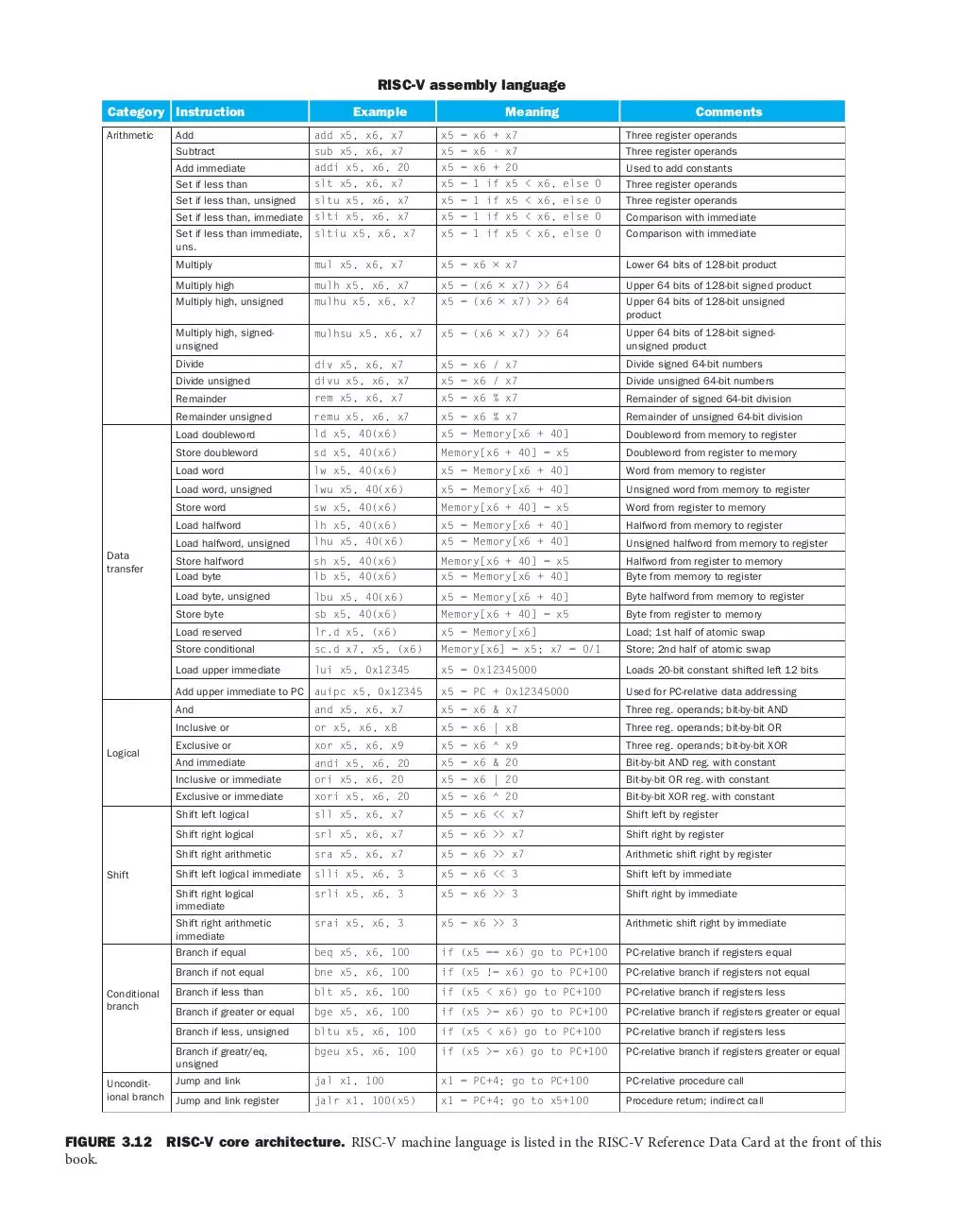# riscvinstructions .pdf

### File information

This PDF 1.7 document has been generated by PDFium, and has been sent on pdf-archive.com on 20/03/2018 at 04:23, from IP address 71.205.x.x. The current document download page has been viewed 237 times.
File size: 54.95 KB (1 page).
Privacy: public file

### Document preview

RISC-V assembly language
Category Instruction
Arithmetic

Data
transfer

Example

Shift

Conditional
branch

Unconditional branch

sub x5, x6, x7

x5 = x6 + x7
x5 = x6 - x7

Three register operands

Subtract

x5 = x6 + 20

Set if less than

slt x5, x6, x7

x5 = 1 if x5 &lt; x6, else 0

Three register operands

Set if less than, unsigned

sltu x5, x6, x7

x5 = 1 if x5 &lt; x6, else 0

Three register operands

Set if less than, immediate

slti x5, x6, x7

x5 = 1 if x5 &lt; x6, else 0

Comparison with immediate

Set if less than immediate,
uns.

sltiu x5, x6, x7

x5 = 1 if x5 &lt; x6, else 0

Comparison with immediate

Three register operands

Multiply

mul x5, x6, x7

x5 = x6 × x7

Lower 64 bits of 128-bit product

Multiply high

mulh x5, x6, x7

x5 = (x6 × x7) &gt;&gt; 64

Upper 64 bits of 128-bit signed product

Multiply high, unsigned

mulhu x5, x6, x7

x5 = (x6 × x7) &gt;&gt; 64

Upper 64 bits of 128-bit unsigned
product

Multiply high, signedunsigned

mulhsu x5, x6, x7

x5 = (x6 × x7) &gt;&gt; 64

Upper 64 bits of 128-bit signedunsigned product

Divide

div x5, x6, x7

x5 = x6 / x7

Divide signed 64-bit numbers

Divide unsigned

divu x5, x6, x7

x5 = x6 / x7

Divide unsigned 64-bit numbers

Remainder

rem x5, x6, x7

x5 = x6 % x7

Remainder of signed 64-bit division

Remainder unsigned

remu x5, x6, x7

x5 = x6 % x7

Remainder of unsigned 64-bit division

ld x5, 40(x6)

x5 = Memory[x6 + 40]

Doubleword from memory to register

Store doubleword

sd x5, 40(x6)

Memory[x6 + 40] = x5

Doubleword from register to memory

lw x5, 40(x6)

x5 = Memory[x6 + 40]

Word from memory to register

lwu x5, 40(x6)

x5 = Memory[x6 + 40]

Unsigned word from memory to register

Store word

sw x5, 40(x6)

Memory[x6 + 40] = x5

Word from register to memory

lh x5, 40(x6)

x5 = Memory[x6 + 40]

Halfword from memory to register

lhu x5, 40(x6)

x5 = Memory[x6 + 40]

Unsigned halfword from memory to register

Store halfword

sh x5, 40(x6)
lb x5, 40(x6)

Memory[x6 + 40] = x5
x5 = Memory[x6 + 40]

Halfword from register to memory
Byte from memory to register

lbu x5, 40(x6)

x5 = Memory[x6 + 40]

Byte halfword from memory to register

Store byte

sb x5, 40(x6)

Memory[x6 + 40] = x5

Byte from register to memory

lr.d x5, (x6)

x5 = Memory[x6]

Load; 1st half of atomic swap

Store conditional

sc.d x7, x5, (x6)

Memory[x6] = x5; x7 = 0/1

Store; 2nd half of atomic swap

lui x5, 0x12345

x5 = 0x12345000

Loads 20-bit constant shifted left 12 bits

x5 = PC + 0x12345000

Three reg. operands; bit-by-bit AND

Add upper immediate to PC auipc x5, 0x12345

Logical

Meaning

And

and x5, x6, x7

x5 = x6 &amp; x7

Inclusive or

or x5, x6, x8

x5 = x6 | x8

Three reg. operands; bit-by-bit OR

Exclusive or

xor x5, x6, x9

x5 = x6 ^ x9

Three reg. operands; bit-by-bit XOR

And immediate

andi x5, x6, 20

x5 = x6 &amp; 20

Bit-by-bit AND reg. with constant

Inclusive or immediate

ori x5, x6, 20

x5 = x6 | 20

Bit-by-bit OR reg. with constant

Exclusive or immediate

xori x5, x6, 20

x5 = x6 ^ 20

Bit-by-bit XOR reg. with constant

Shift left logical

sll x5, x6, x7

x5 = x6 &lt;&lt; x7

Shift left by register

Shift right logical

srl x5, x6, x7

x5 = x6 &gt;&gt; x7

Shift right by register

Shift right arithmetic

sra x5, x6, x7

x5 = x6 &gt;&gt; x7

Arithmetic shift right by register

Shift left logical immediate

slli x5, x6, 3

x5 = x6 &lt;&lt; 3

Shift left by immediate

Shift right logical
immediate

srli x5, x6, 3

x5 = x6 &gt;&gt; 3

Shift right by immediate

Shift right arithmetic
immediate

srai x5, x6, 3

x5 = x6 &gt;&gt; 3

Arithmetic shift right by immediate

Branch if equal

beq x5, x6, 100

if (x5 == x6) go to PC+100

PC-relative branch if registers equal

Branch if not equal

bne x5, x6, 100

if (x5 != x6) go to PC+100

PC-relative branch if registers not equal

Branch if less than

blt x5, x6, 100

if (x5 &lt; x6) go to PC+100

PC-relative branch if registers less

Branch if greater or equal

bge x5, x6, 100

if (x5 &gt;= x6) go to PC+100

PC-relative branch if registers greater or equal

Branch if less, unsigned

bltu x5, x6, 100

if (x5 &lt; x6) go to PC+100

PC-relative branch if registers less

Branch if greatr/eq,
unsigned

bgeu x5, x6, 100

if (x5 &gt;= x6) go to PC+100

PC-relative branch if registers greater or equal

jal x1, 100

x1 = PC+4; go to PC+100

PC-relative procedure call

jalr x1, 100(x5)

x1 = PC+4; go to x5+100

Procedure return; indirect call

FIGURE 3.12  RISC-V core architecture. RISC-V machine language is listed in the RISC-V Reference Data Card at the front of this
book.riscvinstructions.pdf (PDF, 54.95 KB)

### Share on social networks

#### HTML Code

Copy the following HTML code to share your document on a Website or Blog